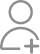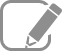# At present , the ratio between the ages of P and Q is 5:7 respectively. If the difference between Q's present age and P's age after 6years is 2 ,what is the total age of P's and Q's present age

Learn Mental Arithmetic +1Follow 139AnswerI'm looking for students who wish to opt for online classes for math-any syllabus (up to 12th grade)

Let the age of P =5x and age of Q=7x Age of P after 6 years =(5x+6) 7x-(5x+6)=2 7x-5x-6=2 2x-6=2 2x=2+6 2x=8 X=8/2=4 P=5x=5*4=20 years Q=7x=7*4=28 years P+Q=20+28=48

I will paint your white Dreams !

I have 9 years of teaching experience in School.

Let's assume P and Q's present ages are pp and qq So p:q=5:7p:q=5:7 ie p/q=5/7p/q=5/7 ie p=5/7×qp=5/7×q As per second statement q−(p+6)=2q−(p+6)=2 {Replace p's value from eqn 1 into eqn 2} q−(5/7×q+6)=2q−(5/7×q+6)=2 ie 2/7×q=82/7×q=8 ie...

Let's assume P and Q's present ages are pp and qq

So p:q=5:7p:q=5:7 ie p/q=5/7p/q=5/7 ie p=5/7×qp=5/7×q

As per second statement

q(p+6)=2q−(p+6)=2

{Replace p's value from eqn 1 into eqn 2}

q(5/7×q+6)=2q−(5/7×q+6)=2

ie 2/7×q=82/7×q=8

ie q=8×7/2=28q=8×7/2=28

So p=20p=20

So total of their present age is 48.

Mathematics teacher with 20 years of experience

Sum of ages =10+14 24

I'm a tutor with ten years of experience

48 years

12

Given , the ratio between the ages of P and Q is 5:7 respectively.=7x-(5x+6x)=2 =X=4 ,sum of their present age =5x+7x =12x =12×4=48 years

"Experienced and qualified teacher and tutor with more than 15 years of experience."

Chemistry Teacher with 2 year of experience

P's present age -20 and Q's present age -28, Total age of P's and Q's present age is - 20+28 = 48.

Total age of p is 5 year Present age of q is 7 year

Now ask question in any of the 1000+ Categories, and get Answers from Tutors and Trainers on UrbanPro.com

Related Lessons

Mastering Simple Calculations, my way!
Did you ever wonder how you can master simple calculations? 37 + 49? let's add 3 to 37 making it 40 and then subtracting 3 from 49 making it 46. Here it is 46 + 40 = 86 which is same as 37 + 49 = 86. ...Looking to Learn?

Find best Tutors and Coaching Centers near you on UrbanPro.

Are you a Tutor or Training Institute?

Join UrbanPro Today to find students near you
X

### Find Tutors, Trainers & Institutes near you

Post requirement and connect with the tutors in your locality

• Select the best Tutor
• Book & Attend a Free Demo
• Pay and start Learning### Want to learn something New?

Find best tutors, trainers & institutes near you on UrbanPro